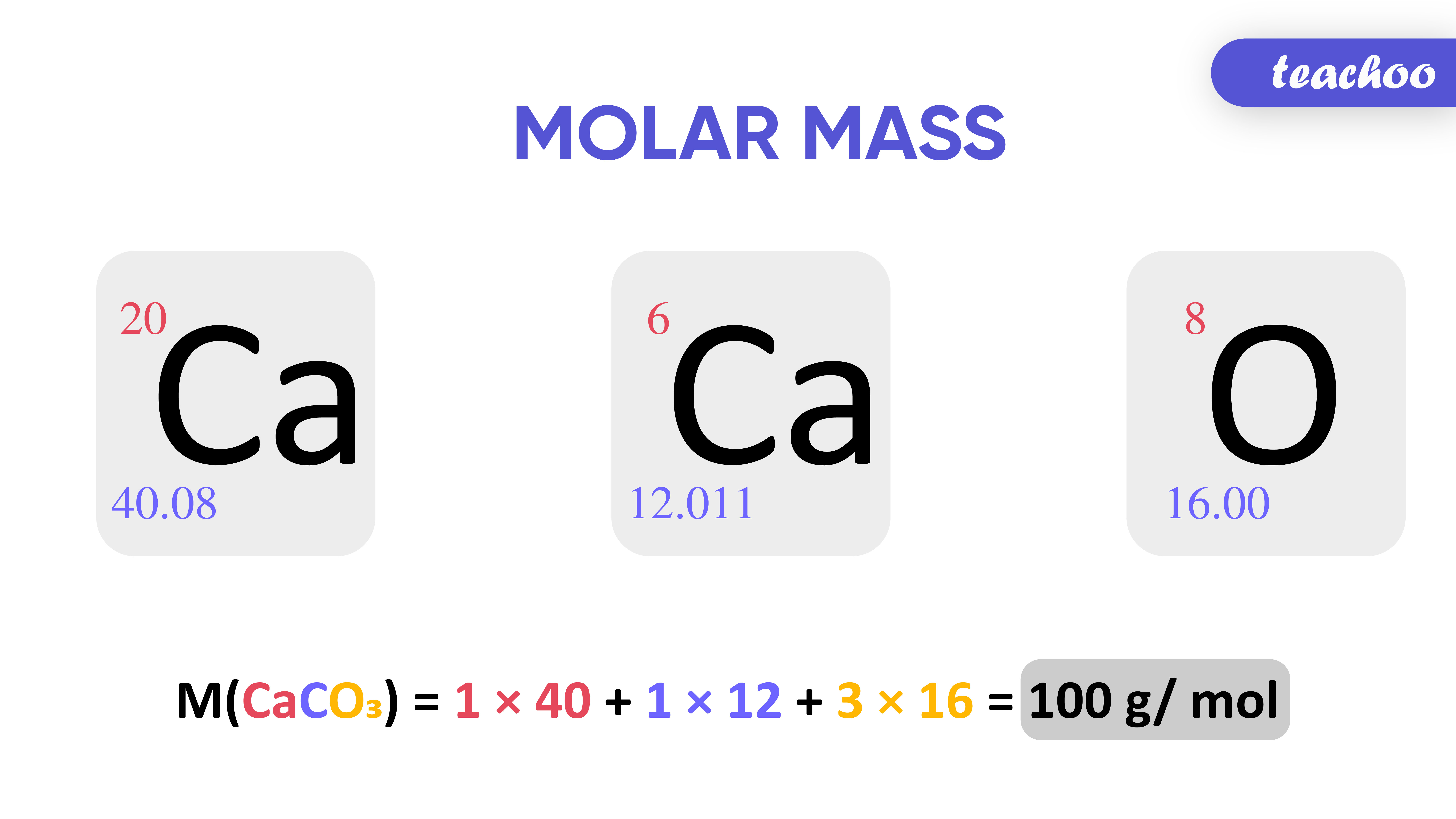Mass of 1 Mole of atom is called Molar Mass

## How is Molar Mass Calculated?

The molar mass of a substance is equal to the atomic mass of a substance taken in grams .

Molar mass is the mass of 1 mole of a substance .

It is denoted by the letter M .

Let us calculate the Molar Mass of Calcium Carbonate (CaCO 3 )## What is a Molecule?

A group of atoms chemically bonded together is Here are the valency of a few elements:
called Molecule

1 molecule of CaCO 3 consists of : 1 Calcium (Ca) atom

1 Carbon (C ) atom

3 Oxygen (O) atoms

M of CaCO 3 = (1 x mass of Ca) + (1 x mass of C) + (3 x mass of O)

M of CaCO 3 = (1 x 40) + (1 x 12) + (3 x 16)

M of CaCO 3 = (1 x 40) + (1 x 12) + (3 x 16)

M of CaCO 3 = 100g/mol

## Example 1

Atomic Mass of hydrogen = 1 u

Hence, Gram Atomic Mass = 1 gram

It means that Mass of 1 mole of Hydrogen = 1 gram

Since 1 MOLE = 6.022 x 10 23  atoms

We can say that

Mass of  6.022 x 10 23  atoms of Hydrogen = 1 gram/mole

## What is a Molecule?

A group of atoms chemically bonded together is Here are the valency of a few elements:
called Molecule

## Example 2

Atomic Mass of oxygen atom = 16 u

Hence, Gram Atomic Mass = 16 gram

It means that Mass of 1 mole of Oxygen = 16 grams

Since 1 MOLE = 6.022 x 10 23  atoms

We can say that

Mass of  6.022 x 10 23  atoms of Oxygen = 16 grams/mole

## Some formulae related to mole

1 mole of an atom = 6.022 x 10 23 atoms = molar mass of that atom

That is,

1 mole of C (Carbon) = 6.022 x 10 23 atoms of C (Carbon) = 12g of Carbon

## What is a Molecule?

A group of atoms chemically bonded together is Here are the valency of a few elements:
called Molecule

Sometimes we're given the values of 2 things and are supposed to find the value of the third.

So, we should remember that,

FORMULA 1 - Number of moles of a substance = Given mass of the substance / Molar mass of the substance

= m/M

Q     Find number of moles of H 2 in 10g of H 2

Ans  Given mass of H 2 = m =10g

Molar Mass of H 2 = M=  2g/mol

We know that,

Number of moles of a substance = Given mass of the substance / Molar mass of the substance

= m/M

= 10g / 2g mol -1

= 5 moles

## What is a Molecule?

A group of atoms chemically bonded together is Here are the valency of a few elements:
called Molecule

1.         How many moles are there in 52g of Helium?

We know that

Atomic Mass of Helium = 4 u

So Molar Mass of  Helium = 4 g mol -1

It means that Mass of 1 mole of Helium = 4 grams

We can also write as

= m/M

= 52/4

=13

So, 13 moles are there in 52g of Helium

FORMULA 2 - Number of moles of a substance = Number of particles of the substance / Avogadro's Constant

= Number of particles / 6.022 x 10 23

= N/N O

1. How many moles is 12.044 x 10 23 atoms of He?

A Number of atoms of the He = N = 12.044 x 10 23

## What is a Molecule?

A group of atoms chemically bonded together is Here are the valency of a few elements:
called Molecule

Avogadro's Constant = N O = 6.022 x 10 23

We know that,

Number of moles of a substance = Number of particles of the substance / Avogadro's Constant

= N/N O

= 11.044 x 10 23 / 6.022 x 10 23

=  2 moles of He atoms

Note - We usually denote number of moles by 'n'

So, this brings us to the 3rd formula;

FORMULA 3 -  Given mass of the substance / Molar mass of the substance = Number of particles of the substance / Avogadro's Constant

=> m/M = N/N O

Example - 3.3, 3.4

In text Question -

NCERT Question -7,8,9,10,11

## What is a Molecule?

A group of atoms chemically bonded together is Here are the valency of a few elements:
called Molecule

1. Class 9
2. Chapter 3 Class 9 - Atoms And Molecules (Term 2)
3. Concepts

Concepts

Class 9
Chapter 3 Class 9 - Atoms And Molecules (Term 2)